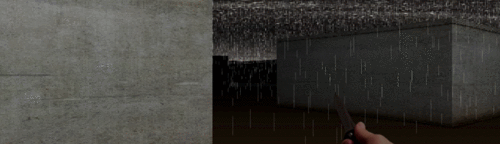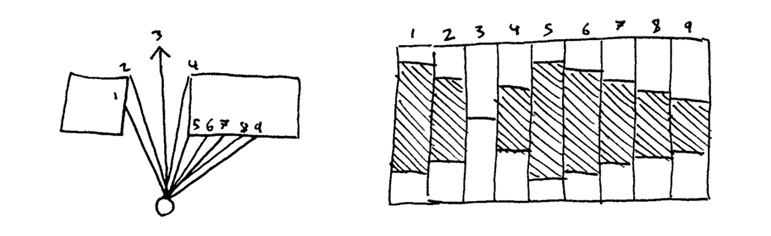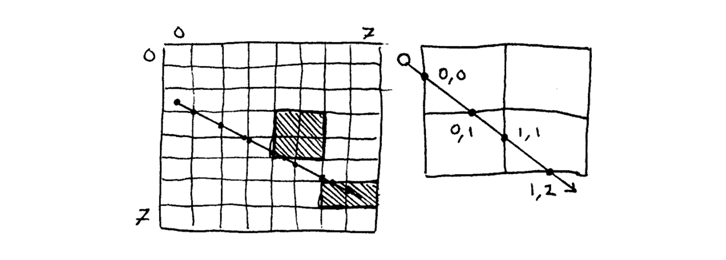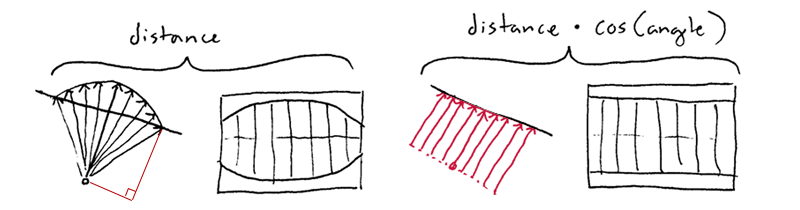# 265行代码实现第一人称引擎

A very small man can cast a very large shadow.

Varys## 玩家

``````function Player(x, y, direction) {
this.x = x;
this.y = y;
this.direction = direction;
}``````

## 地图

``````function Map(size) {
this.size = size;
this.wallGrid = new Uint8Array(size * size);
}``````

## 投射一束光线1.找到每条光线的角度

``````var angle = this.fov * (column / this.resolution - 0.5);
var ray = map.cast(player, player.direction + angle, this.range);``````

2.通过网格跟踪每条光线``````function ray(origin) {
var stepX = step(sin, cos, origin.x, origin.y);
var stepY = step(cos, sin, origin.y, origin.x, true);
var nextStep = stepX.length2 < stepY.length2
? inspect(stepX, 1, 0, origin.distance, stepX.y)
: inspect(stepY, 0, 1, origin.distance, stepY.x);

if (nextStep.distance > range) return [origin];
return [origin].concat(ray(nextStep));
}``````

``````var dx = run > 0 ? Math.floor(x + 1) - x : Math.ceil(x - 1) - x;
var dy = dx * (rise / run);``````

3.绘制一列

``````var z = distance * Math.cos(angle);
var wallHeight = this.height * height / z;``````## 渲染出来

``````Camera.prototype.render = function(player, map) {
this.drawSky(player.direction, map.skybox, map.light);
this.drawColumns(player, map);
this.drawWeapon(player.weapon, player.paces);
};``````

• 分辨率决定了每帧要画多少列，即要投射多少条光线。
• 视野决定了我们能看的宽度，即光线的角度。
• 射程决定了我们能看多远，即光线长度的最大值

## 组合起来

``````oop.start(function frame(seconds) {
map.update(seconds);
player.update(controls.states, map, seconds);
camera.render(player, map);
});``````

## 细节

### 雨滴

``````var rainDrops = Math.pow(Math.random(), 3) * s;
var rain = (rainDrops > 0) && this.project(0.1, angle, step.distance);

ctx.fillStyle = '#ffffff';
ctx.globalAlpha = 0.15;
while (--rainDrops > 0) ctx.fillRect(left, Math.random() * rain.top, 1, rain.height);``````

### 照明和闪电

``````ctx.fillStyle = '#000000';
ctx.globalAlpha = Math.max((step.distance + step.shading) / this.lightRange - map.light, 0);
ctx.fillRect(left, wall.top, width, wall.height);``````

### 碰撞检测

``````Player.prototype.walk = function(distance, map) {
var dx = Math.cos(this.direction) * distance;
var dy = Math.sin(this.direction) * distance;
if (map.get(this.x + dx, this.y) <= 0) this.x += dx;
if (map.get(this.x, this.y + dy) <= 0) this.y += dy;
};``````

### 墙壁贴图

``````step.offset = offset - Math.floor(offset);
var textureX = Math.floor(texture.width * step.offset);``````

## 接下来做什么？

• 浸入式体验。样例在求你为它加上全屏、鼠标定位、下雨背景和闪电时同时出现雷响。
• 室内级别。用对称渐变取代天空盒。或者，你觉得自己很屌的话，尝试用瓷片渲染地板和天花板。（可以这么想：所有墙面画出来之后，画面剩下的空隙就是地板和天花板了）
• 照明对象。我们已经有了一个相当健壮的照明模型。为何不将光源放到地图上，通过它们计算墙的照明？光源占了80%大气层。
• 良好的触摸事件。我已经搞定了一些基本的触摸操作，手机和平板的小伙伴们可以尝试一样 demo。但这里还有巨大的提升空间。
• 摄像机特效。比如放大缩小、模糊、醉汉模式等等。有了光线投射器这些都显得特别简单。先从控制台中修改 camera.fov 开始。

Hacker News 上的讨论